# Operators and Expressions in C++

## Operators and Expressions in C++

In this article, I am going to discuss Operators and Expressions in C++ with examples. In our previous article, I introduced you to the basics of programming including basic welcome to C++ program, Data types, and variables. In this article, we are also continuing with C++ programming basics operators and expressions. The topics discussed in this article are as follows:

1. Operators
2. Arithmetic operators
3. Operator Precedence and Associativity
4. Compound Assignment Operator
5. Increment/Decrement Operator
6. Bitwise Operators
##### Operators in C++:

Operators are predefined in C++ and it is used to perform a computational task. Usually, operators are symbols. The C++ built-in operators are as follows.

1. Arithmetic operators
2. Relational operators
3. Logical operators
4. Bitwise operators
5. Assignment Operators
##### Arithmetic Operators in C++:

The operators that are used to perform mathematical operations are called Arithmetic operators. Symbols are similar to the one which we used in mathematics.

1. Addition of number is done using ‘+’
2. Subtraction ‘-‘
3. Multiplication ‘*’ (asterisk) (note: In mathematics we use x but in C++ it is an asterisk.)
4. Division ‘/’
5. Modulus ‘%’ (to identify the remainder or to find modulo)
6. Increment Operator ++ (adds one to an already existing value or variable)
7. Decrement Operator — (subtract one from an already existing value)

Note: Increment or decrement operators are usually use in loops, which will be discussed later.

##### Example:

Let us write a program, which uses all the arithmetic operators.

```#include <iostream>
using namespace std;
int main ()
{
int no1, no2;
cout << "enter the values for number 1 and number2 \n";
cin >> no1 >> no2;//to read the value of two number

cout << "Addtion of two numbers:\t" << no1 + no2 << endl;
cout << "Subtraction of two numbers:\t" << no1 - no2 << endl;
cout << "Multiplication of two numbers:\t" << no1 * no2 << endl;

//note we should no pass no2 value as zero.input validation needs to be done
//but will discuss in later section after discussion conditional statements
cout << "Division of two numbers:\t" << no1 / no2 << endl;
cout << "Modulus of two numbers:\t" << no1 % no2 << endl;

//note there is preincrement/decrement and post increment/decrement
//which will be discuss later in this section
cout << "Increment no1:\t" << ++no1 << endl;
cout << "Decrement  no1:\t" << --no1 << endl;

return 0;
}

```

Output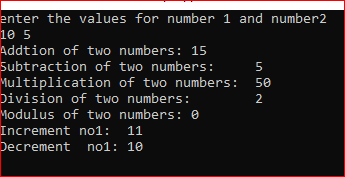##### Arithmetic Precedence and Expression in C++

Before explaining Precedence, let us understand the expression. The expression is just a combination of variables along with operators.

Example: a+b; here a+b is an expression. It is an algebraic expression.

Precedence: precedence is nothing but the priority of evaluating the expression when two or more operators are there.

Example: let’s consider an expression a+b*c where a=5, b=6, and c=7. Can you tell me the output of a+b*c?

Without considering precedence. If we evaluate the expression then we end up in the wrong value. In the above example without precedence values is 77.

##### How?

a+b I will add first which results in 11. Then I will multiply it with c resulting in 77 which is wrong. With precedence, the Answer would be different.

b*c will evaluate first as multiplication as more precedence than addition. So, b*c result in 42. Then addition will be performed 42+5 results in 47.

##### Let see the same output in the code.
```#include <iostream>
using namespace std;
int main ()
{
int a = 5, b = 6, c = 7;
cout << "result:" << a + b * c << endl;
return 0;
}
```

Output: result:47

##### Operator Precedence in C++

Now let us see the precedence of arithmetic operators. Here currently we are discussing only the precedence of arithmetic operators and relational, logical, and bitwise operators also have precedence that will be discussed later.

 Operators Assumed Precedence (note higher the number more the priority or precedence) (),++,–(post-increment or decrement) 3 *,/,% 2 +,- (pre-increment or decrement) 1

From this, we came to know addition and subtraction as the same precedence and multiplication division and modulus as the same precedence.

##### Operator Associativity:

To explain this concept, I will directly go for example. Let’s say I have an expression a*b/c where a=8, b=9, and c=10. Can you tell me the output?

Since division and multiplication have the same precedence there could be two outputs.1

1. 8*9/10= 7 //here 8*9 multiplied first then division happens
2. 8*9/10= 0 if the result is an integer. Here division then multiplication.

To solve this conflict operator associativity comes into the picture. This tells the compiler from where operators’ evaluation needs to be done whether it is from the left or from right based on operator associativity. In our example, multiplication and division are left to right associativity. So that output will be 7.

```#include <iostream>
using namespace std;
int main ()
{
int a = 8, b = 9, c = 10;
cout << "result:" << a * b / c << endl;
return 0;
}
```

Output: result:7

So, if we update the above table.

 Operators Assumed Precedence (note higher the number more the priority or precedence) Operator Associativity (), ++, — (post-increment or decrement) 3 Left-to-right *,/,% 2 Left-to-right +,- 1 Left-to-right ++, — (pre-increment or decrement) 1 Right to Left
##### Assignment for you:

Please try to solve the below Assignments on Arithmetic Operators, Precedence and Associativity. The solution will be given in this article. please see it in case if you were stuck.

1. Program to find the area of a rectangle.
2. Program to calculate the Simple interest.
##### Compound Assignment Operator in C++:

Compound Assignment is there not only for arithmetic operators but it is there for other operators also. However, we will discuss compound assignment arithmetic operator later whenever we discuss those operators at that time, I will discuss the compound operators also.

Compound assignment operator usually does the same thing what existing operators are doing but it gives more efficient in compile time.

To explain compound assignment Operator let us take an example: I have a variable I want to repeated add another no to this variable. Currently, without compound assignment operator I am following this method.

I am writing a pseudocode

int sum=0;
Sum=sum+5;
Sum=sum+8;
Sum=sum+11; //here every time you are adding sum with other values and storing back it in sum only.

The Variable sum is evaluated in each instruction, which consumes more compile-time hence inefficient. To overcome this problem and also to increase program readability compound assignment operators or shorthand operators come into the picture. Now the same pseudo-code can be written as

int sum=0;
Sum+=5;
Sum+=8;
Sum+=11;

Let’s see how efficient it is by comparing the compile time for both.

##### Without shorthand operator/compound assignment operator

Compile-time is 4.119 seconds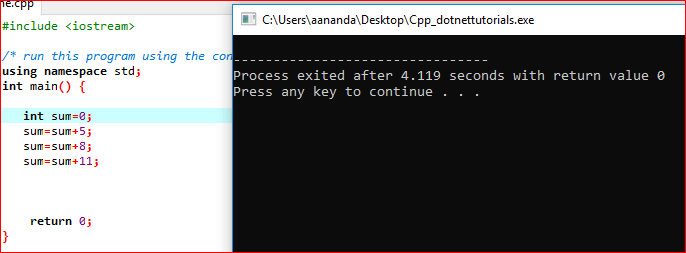##### With shorthand operator/compound assignment operator

Compile-time is only 1.788 seconds.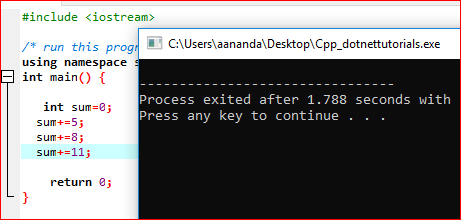The compound assignment operators are. +=,-=,*=,/=,%=.

Note: Assignment and Compound Assignment Operators have the least precedence when compare to other arithmetic operators.

##### Increment/Decrement Operator:

Increment or Decrement operator will increment/ Decrement the variable value and assign it back the variable.

Example: int i=5; i++; here value of i now changed to 6 which is similar to i=i+1;

Intern similar to i+=1; all give the same meaning.

Increment operators are widely used for counting purposes. Especially in loops. There are two types of increment/decrement operators.

1. Post increment/decrement
2. Pre increment/decrement

To understand the difference between these two lets us take a look at the following example.
int a=10, b;
b=a++; //post-increment
if I print this value a is now 11 but b is 10.
Because post-increment increments the values after the line execution.
Means after assigning value to b.

int a=10, b;
b=++a; //pre-increment
This prints a and b value as 11 because the value got increment before assigning it to b.

Note. We should not use multiple ++/– on the same variable in a single expression. Because the result will become unpredictable.

Example: int i=10, j; j=++i + i++ + i++; //here we can’t say what the result unless we run the program.

##### Efficient of Increment/decrement operator over shorthand operator.

Compile-time is 0.998 seconds.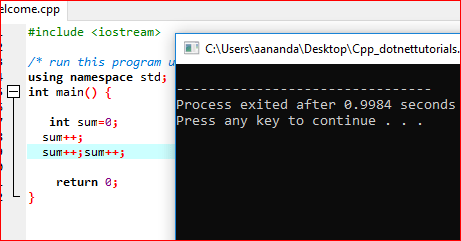##### Bitwise Operators in C++:

Bitwise operators operate on bits and it performs a bit-by-bit operation. The Bitwise operators in C++ are as follows.

1. Bitwise And &
2. Bitwise OR |
3. Bitwise X-OR ^
4. Bitwise Not ~
5. Binary Leftshift <<
6. Binary Rightshift >>

If we take two bits bit1 and bit2 then the truth table for these operators are

##### Bitwise And
 Bit1 Bit2 Bit1&Bit2 0 0 0 0 1 0 1 0 0 1 1 1
##### Bitwise OR
 Bit1 Bit2 Bit1 | Bit2 0 0 0 0 1 1 1 0 1 1 1 1
##### Bitwise XOR
 Bit1 Bit2 Bit1 ^ Bit2 0 0 0 0 1 1 1 0 1 1 1 0

Assume A=10 and B=13 then the value of bitwise and or operation is as follows.

A= 0000 1010
B= 0000 1101
A&B 0000 1000
A |B 0000 1110
A^B 0000 0110
~A 1000 1001

Binary leftshift shifts the bits to the left side as the number of times you specified. Mainly it will do multiplication. Binary RightShift shifts the bits to the Right side as the number of times you specified. Mainly it will do division.

##### This is how the Bitwise operators work.
```#include <iostream>
using namespace std;
int main ()
{
int a = 10, b = 13;
cout << "Bitwise AND: \t" << (a & b) << endl;
cout << "Bitwise OR: \t" << (a | b) << endl;
cout << "Bitwise X-OR: \t" << (a ^ b) << endl;
cout << "Bitwise NOt A: \t" << ~a << endl;
cout << "Bitwise Not B: \t" << ~b << endl;
a = a << 2;
cout << "Bitwise leftshift of a:\t" << a << endl;
b = b >> 2;
cout << "Bitwise Rightshift of b:\t" << b << endl;
return 0;
}
```

Output: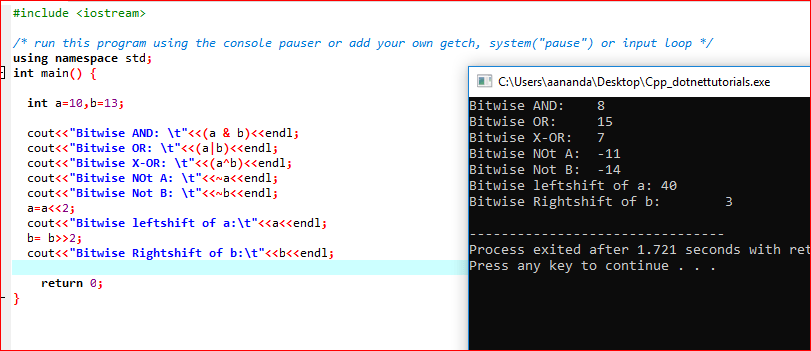In the next article, I will start the conditional statement where I am going to introduce you to the logical and relational operators. Here, in this article, I try to explain Operators and Expressions in C++ with examples. I hope you enjoy these Operators and Expressions in C++ with examples article.